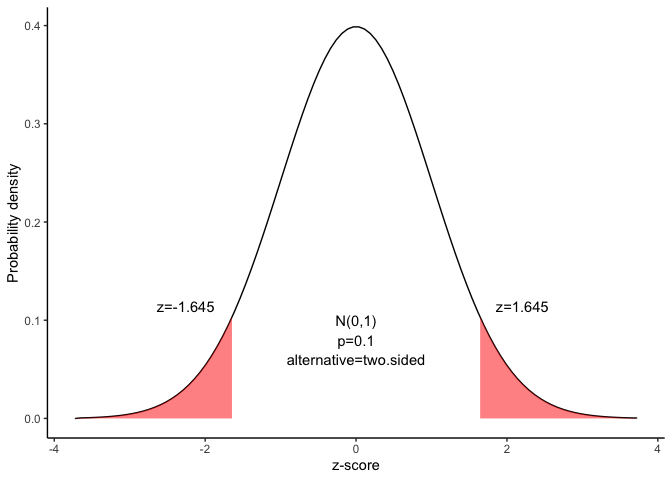# Confidence interval for the difference between proportions

This document is prepared automatically using the following R command.

 library(interpretCI)x=propCI(n1 = 400, n2 = 300, p1 = 0.4, p2 = 0.3, alpha = 0.1)interpret(x)

## Problem

 Suppose the Cartoon Network conducts a nation-wide survey to assess viewer attitudes toward Superman. Using a simple random sample, they select 400 boys and 300 girls to participate in the study. Forty percent of the boys say that Superman is their favorite character, compared to thirty percent of the girls. What is the 90% confidence interval for the true difference in attitudes toward Superman?

## Solution

The approach that we used to solve this problem is valid when the following conditions are met.

• The sampling method must be simple random sampling. This condition is satisfied; the problem statement says that we used simple random sampling.

• Both samples should be independent. This condition is satisfied since neither sample was affected by responses of the other sample.

• The sample should include at least 10 successes and 10 failures. Suppose we classify choosing Superman as a success, and any other response as a failure. Then, we have plenty of successes and failures in both samples.

• The sampling distribution should be approximately normally distributed. Because each sample size is large, we know from the central limit theorem that the sampling distribution of the difference between sample proportions will be normal or nearly normal; so this condition is satisfied.

## Solution

Since the above requirements are satisfied, we can use the following four-step approach to construct a confidence interval.

### 1. Identify a sample statistic.

Since we are trying to estimate the difference between population proportions, we choose the difference between sample proportions as the sample statistic. Thus, the sample statistic is $$p_{boy} - p_{girl} = 0.4 - 0.3 = 0.1$$.

### 2. Select a confidence level.

In this analysis, the confidence level is defined for us in the problem. We are working with a 90% confidence level.

### 3. Find the margin of error.

#### Find standard deviation or standard error.

Since we do not know the population proportions, we cannot compute the standard deviation; instead, we compute the standard error. And since each population is more than 20 times larger than its sample, we can use the following formula to compute the standard error (SE) of the difference between proportions:

$SE= \sqrt{\frac{p_1(1-p_1)}{n_1}+\frac{p_2(1-p_2)}{n_2}}$ where $$p_1$$ is the sample proportion for sample 1, $$n_1$$ is the sample size from population 1, $$p_2$$ is the sample proportion for sample 2 and $$n_2$$ is the sample size from population 2.

$SE= \sqrt{\frac{0.4(1-0.4)}{400}+\frac{0.3(1-0.3)}{300}}=0.036$

Find the critical probability(p*):

$p*=1-\alpha/2=1-0.1/2=0.95$

The critical value is the z statistic having a cumulative probability equal to 0.95.

We can get the critical value using the following R code.

$qnorm(p)=qnorm(0.95)=1.645$

Alternatively, we find that the critical value is 1.645 from the normal Distribution table.

 alpha 0.4 0.25 0.1 0.05 0.025 0.01 0.005 0.001 z -0.253 -0.674 -1.282 -1.645 -1.96 -2.326 -2.576 -3.09

The graph shows the $$\alpha$$ values are the tail areas of the distribution.Compute margin of error(ME):

$ME=critical\ value \times SE$ $ME=1.645 \times 0.036=0.059$

Specify the confidence interval. The range of the confidence interval is defined by the sample statistic $$\pm$$margin of error. And the uncertainty is denoted by the confidence level.

### 4. Confidence interval of the proportion

Therefore, the 90% confidence interval is 0.04 to 0.16. That is, we are 90% confident that the true proportion is in the range 0.04 to 0.16. Since both ends of the confidence interval are positive, we can conclude that more boys than girls choose Superman as their favorite cartoon character.

### Result of propCI()

$data # A tibble: 1 × 2 x y <lgl> <lgl> 1 NA NA$result
alpha  p1  p2  n1  n2  DF  pd         se critical         ME      lower
1   0.1 0.4 0.3 400 300 698 0.1 0.03605551 1.644854 0.05930604 0.04069396
upper                     CI   ppooled   sepooled       z      pvalue
1 0.159306 0.10 [90CI 0.04; 0.16] 0.3571429 0.03659625 2.73252 0.006285182
alternative
1   two.sided

\$call
propCI(n1 = 400, n2 = 300, p1 = 0.4, p2 = 0.3, alpha = 0.1)

attr(,"measure")
 "propdiff"

### Reference

The contents of this document are modified from StatTrek.com. Berman H.B., “AP Statistics Tutorial”, [online] Available at: https://stattrek.com/estimation/difference-in-proportions.aspx?tutorial=AP URL[Accessed Data: 1/23/2022].